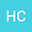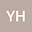Conservative compact difference scheme based on the scalar auxiliary variable method for the generalized Kawahara equation
••Hongtao Chen
Xiamen University

Corresponding Author:[email protected]

Author Profile## Abstract

In this paper, a conservative compact difference scheme for the generalized Kawahara equation is constructed based on the scalar auxiliary variable (SAV) approach. The discrete conservative laws of mass and Hamiltonian energy and boundedness estimates are studied in detail. The error estimates in discrete $L^{\infty}$-norm and $L^2$-norm of the presented scheme are analyzed by using the discrete energy method. We give an efficiently algorithm of the presented scheme which only needs to solve two decoupled equations.
27 Oct 2021Submitted to Mathematical Methods in the Applied Sciences
28 Oct 2021Submission Checks Completed
28 Oct 2021Assigned to Editor
04 Nov 2021Reviewer(s) Assigned
15 Apr 2022Review(s) Completed, Editorial Evaluation Pending
17 Apr 2022Editorial Decision: Revise Major